Math Olympiad Test: Surface Area and Volume- 2

# Math Olympiad Test: Surface Area and Volume- 2

Test Description

## 15 Questions MCQ Test Mathematics Olympiad for Class 9 | Math Olympiad Test: Surface Area and Volume- 2

Math Olympiad Test: Surface Area and Volume- 2 for Class 9 2022 is part of Mathematics Olympiad for Class 9 preparation. The Math Olympiad Test: Surface Area and Volume- 2 questions and answers have been prepared according to the Class 9 exam syllabus.The Math Olympiad Test: Surface Area and Volume- 2 MCQs are made for Class 9 2022 Exam. Find important definitions, questions, notes, meanings, examples, exercises, MCQs and online tests for Math Olympiad Test: Surface Area and Volume- 2 below.
Solutions of Math Olympiad Test: Surface Area and Volume- 2 questions in English are available as part of our Mathematics Olympiad for Class 9 for Class 9 & Math Olympiad Test: Surface Area and Volume- 2 solutions in Hindi for Mathematics Olympiad for Class 9 course. Download more important topics, notes, lectures and mock test series for Class 9 Exam by signing up for free. Attempt Math Olympiad Test: Surface Area and Volume- 2 | 15 questions in 15 minutes | Mock test for Class 9 preparation | Free important questions MCQ to study Mathematics Olympiad for Class 9 for Class 9 Exam | Download free PDF with solutions
 1 Crore+ students have signed up on EduRev. Have you?
Math Olympiad Test: Surface Area and Volume- 2 - Question 1

### The curved surface area and the volume of a pillar are 264m2 and 396m3. What is the height of the pillar?

Detailed Solution for Math Olympiad Test: Surface Area and Volume- 2 - Question 1

Curved surface area of pillar = 264
⇒ 2πrh = 264
Volume of pillar = 396
⇒ πr2h = 396
∴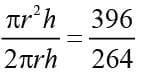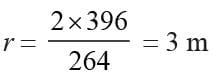∴ 2πrh = 264
Now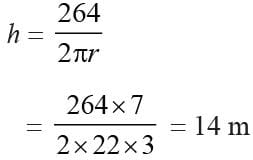Math Olympiad Test: Surface Area and Volume- 2 - Question 2

### The volume of a cone is 1232 cm3 and the diameter of its base is 14cm. What is its slant height?

Detailed Solution for Math Olympiad Test: Surface Area and Volume- 2 - Question 2

The volume of the cone = 1232
⇒ 1/3 πr2h = 1232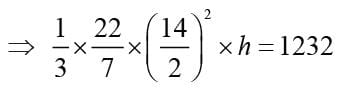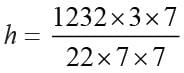= 24 cm
Slant height = l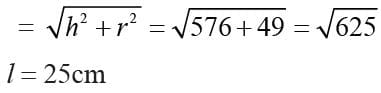Math Olympiad Test: Surface Area and Volume- 2 - Question 3

### The radius of a wire is decreased to one third. If volume remains the same, the length will become how many times?

Detailed Solution for Math Olympiad Test: Surface Area and Volume- 2 - Question 3

Let radius be r and height be h.
New radius be r/3 and height H.
∴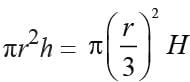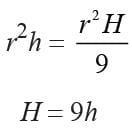Length will become 9 times.

Math Olympiad Test: Surface Area and Volume- 2 - Question 4

The volume of a cube is 512 cm3. What is its surface area?

Detailed Solution for Math Olympiad Test: Surface Area and Volume- 2 - Question 4

Volume of the cube = 512
⇒ a3 = 512 = 83
⇒ a = 8
∴ Total surface area = 6a2 = 6 × 82
= 6 × 64  = 384 cm2

Math Olympiad Test: Surface Area and Volume- 2 - Question 5

A solid metallic cylinder of base radius 3cm and height 5cm is melted to make a solid cone of height 1cm and base radius 1mm. What is the number of cones?

Detailed Solution for Math Olympiad Test: Surface Area and Volume- 2 - Question 5

Number of cones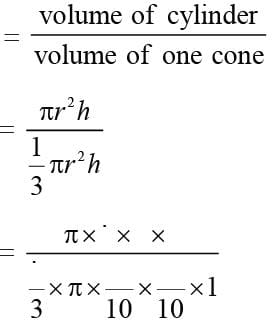= 3 × 3 × 3 × 5 × 10 × 10
= 135 × 100
= 13500

Math Olympiad Test: Surface Area and Volume- 2 - Question 6

A metallic sphere of radius 10.5cm is melted and then recast into small cones each of radius 3.5cm and length 3cm. What is the number of such cones?

Detailed Solution for Math Olympiad Test: Surface Area and Volume- 2 - Question 6

No. of cones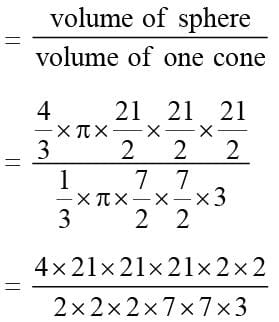= 3 × 21 × 2 = 126

Math Olympiad Test: Surface Area and Volume- 2 - Question 7

A cone and a hemisphere have equal bases and equal volumes. What is the ratio of their heights?

Detailed Solution for Math Olympiad Test: Surface Area and Volume- 2 - Question 7

Cone and hemisphere have equal base means equal radii, of R cm,
Height of the cone be H cm
Height of hemisphere = R cm
Volume of cone = volume of hemisphere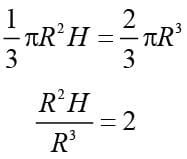H/R = 2/1

Math Olympiad Test: Surface Area and Volume- 2 - Question 8

How many lead shots each 0.3 cm in diameter can be made from a cuboid of dimension 18cm × 22cm × 6cm?

Detailed Solution for Math Olympiad Test: Surface Area and Volume- 2 - Question 8

No. of lead shots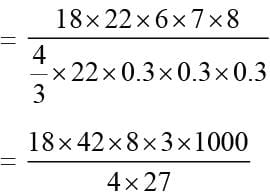= 168000

Math Olympiad Test: Surface Area and Volume- 2 - Question 9

The diameter of a roller 1m long is 84 cm. If it takes 200 complete revolutions to level ground, what is the area of the ground?

Detailed Solution for Math Olympiad Test: Surface Area and Volume- 2 - Question 9

Radius of roller = 84/2 = 42cm
h = 100cm
Area covered by the roller in 200
Revolutions = 200 × 2πrh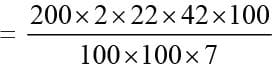= 4 × 22 × 6
= 88 × 6 = 528 m2

Math Olympiad Test: Surface Area and Volume- 2 - Question 10

The curved surface area of a cylindrical pillar is 264 m2 and its volume is 924 m3. What is the height of the pillar?

Detailed Solution for Math Olympiad Test: Surface Area and Volume- 2 - Question 10

Here 2πrh = 264 m2
and  πr2h = 924 m3
∴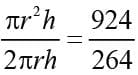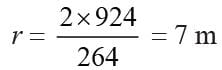∴ Putting    r = 7
2πrh = 264
then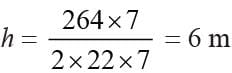Math Olympiad Test: Surface Area and Volume- 2 - Question 11

A small indoor greenhouse is made entirely of glass panes including a base held together with tape. How much tape is needed for all the 12 edges?

Detailed Solution for Math Olympiad Test: Surface Area and Volume- 2 - Question 11

Length of the tape = 4 (l + b + h)
= 4 (30 + 25 + 25)
= 4 × 80 = 320 cm.

Math Olympiad Test: Surface Area and Volume- 2 - Question 12

How many 3 meter cubes can be cut from a cuboid measuring 18 m × 12 m × 9 m?

Detailed Solution for Math Olympiad Test: Surface Area and Volume- 2 - Question 12

Edge of each cube = 3 m
Volume of each cube = (3)3 = 27 m3
Volume of the cuboid = 18 × 12 × 9 = 1944 m3
Therefore, number of cubes = 1944/27​ = 72

Math Olympiad Test: Surface Area and Volume- 2 - Question 13

A rectangular reservoir is 120 m long and 75 m wide. At what speed per hour must water flow into it through a square pipe of 20 cm wide so that the water rises by 2.4 m in 18 hours?

Detailed Solution for Math Olympiad Test: Surface Area and Volume- 2 - Question 13

Volume of the water accumulated the reservoir 18 hours = (120 × 75 × 2.4) m3
Let speed of water = v km/hour.
The width of cuboid = b = 20/100 = 1/5 m
Height = h = 20/100 = 1/5 m
Length of water cuboid formed in 18 hours
= 18 v km = 18 × 1000 v m
= 18000 v m
Volume of the water accumulated in reservoir in 18 hours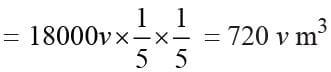⇒ 720 v = 120 × 75 × 2.4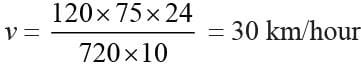Math Olympiad Test: Surface Area and Volume- 2 - Question 14

The thickness of a hollow wooden cylinder is 2cm. It is 35cm long and its inner radius is 12cm. What is the volume of the wood required to make the cylinder if it is open at either end?

Detailed Solution for Math Olympiad Test: Surface Area and Volume- 2 - Question 14

Let r be the inner radius of the cylinder
r = 12cm
Outer radius = R = 12 + 2 = 14cm
h = 35cm
Volume of wood = π (R2 – r2) h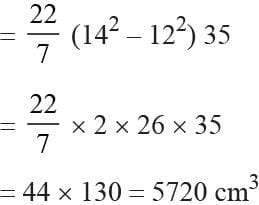Math Olympiad Test: Surface Area and Volume- 2 - Question 15

A solid cylinder has a total surface area of 462 m2. Its curved surface area is one-third of the total surface area. What is the volume of the cylinder?

Detailed Solution for Math Olympiad Test: Surface Area and Volume- 2 - Question 15

Curved surface area
= 1/3 × total surface area
= 1/3 × 462 = 154
⇒ 2πrh = 154 …(1)
Total surface area = 462
⇒ 2πrh + 2πr2 = 462
⇒ 154 + 2πr2 = 462
⇒ 2πr2 = 308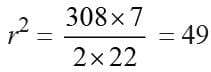Putting this value in (i), we get
2πrh = 154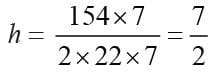∴  Volume of cylinder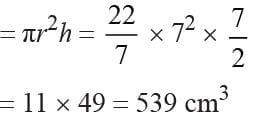## Mathematics Olympiad for Class 9

1 videos|43 tests
 Use Code STAYHOME200 and get INR 200 additional OFF Use Coupon Code
Information about Math Olympiad Test: Surface Area and Volume- 2 Page
In this test you can find the Exam questions for Math Olympiad Test: Surface Area and Volume- 2 solved & explained in the simplest way possible. Besides giving Questions and answers for Math Olympiad Test: Surface Area and Volume- 2, EduRev gives you an ample number of Online tests for practice

## Mathematics Olympiad for Class 9

1 videos|43 tests